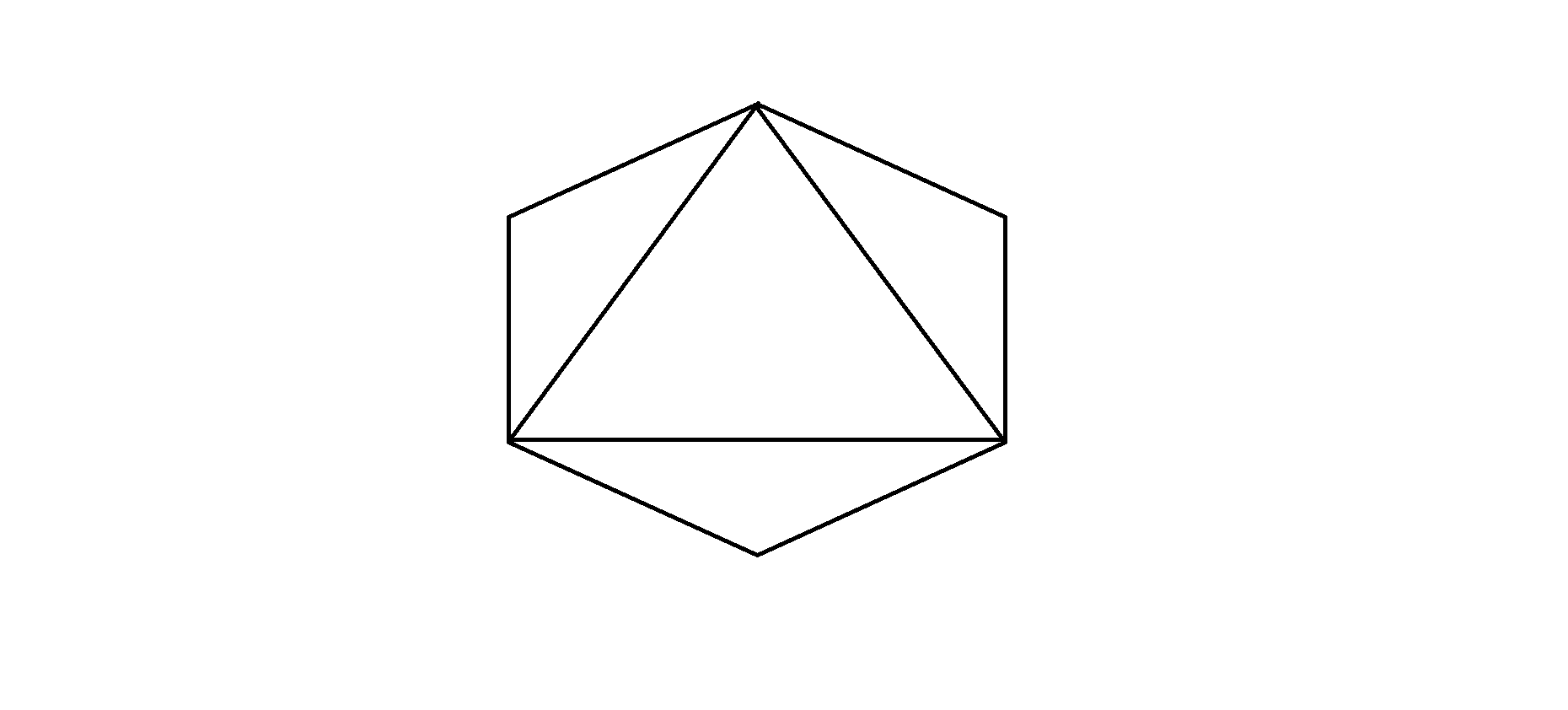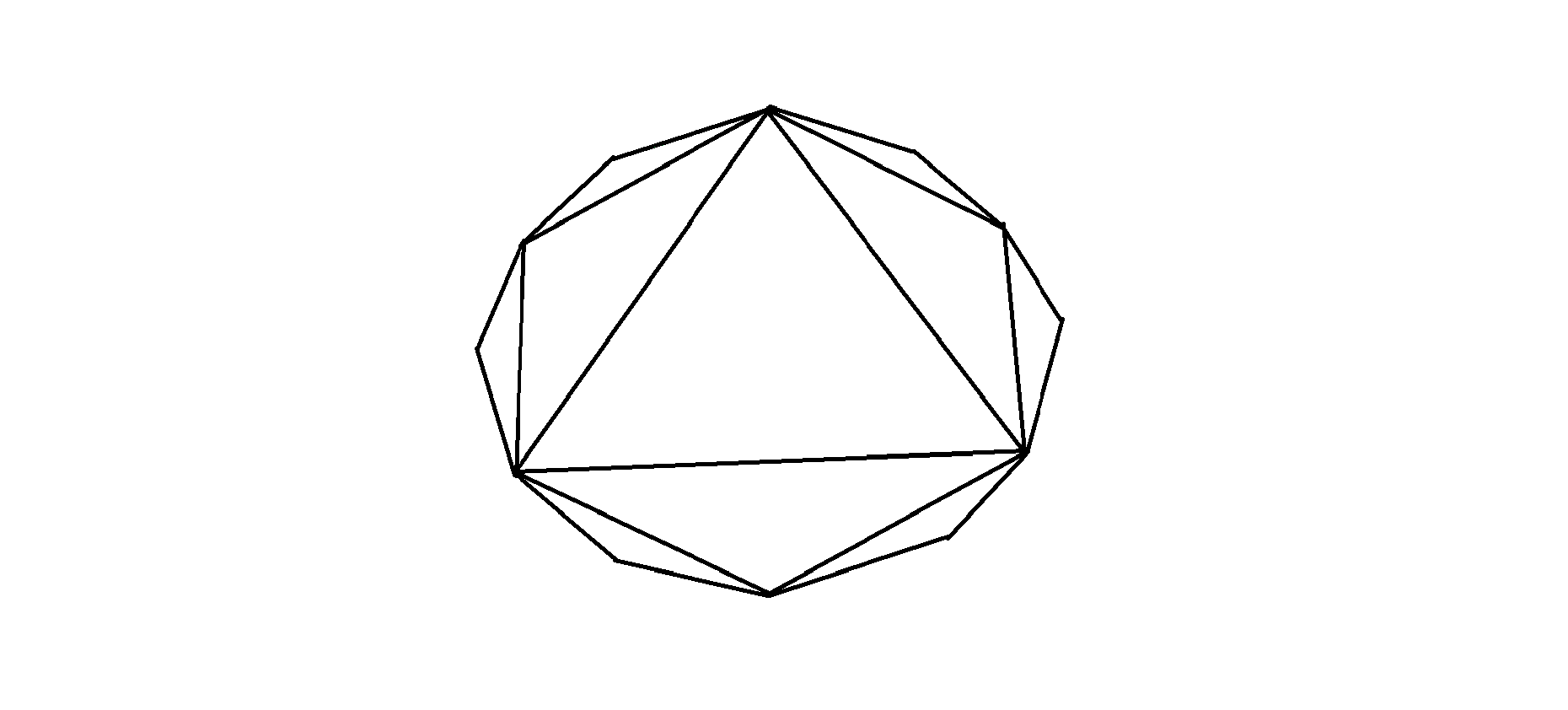# Count of nested polygons that can be drawn by joining vertices internally

• Difficulty Level : Easy
• Last Updated : 07 Apr, 2021

Given a regular polygon with N sides. The task is to find the count of polygons that can be drawn from the given polygon by joining the vertices of the given polygon internally.

Examples:

Input: N = 6
Output: 1
Explanation:There is only one nested polygon i.e., Triangle whose sides are the chords of the immediate parent polygon i.e., Hexagon.

Input: N = 12
Output: 2
Explanation:There are two nested polygons. First one is a Hexagon and second one is a Triangle. The sides of both of the nested polygons are actually chords of their immediate parent polygon.

Approach: To solve this problem observe the following:

1. Polygons with a number of sides less than or equal to 5 can not produce nested polygons, i.e. polygons of sides ≤5 will always have at least one side overlapping with its nested polygon.
2. Each side of a nested polygon takes two consecutive sides of the immediate parent polygon.

With the above observations, it is easy to conclude that number of sides of each nested polygon is half of the number of sides of its immediate parent polygon. So, we keep dividing N by 2, and increment the counter for nested polygons, until N becomes less than or equal to 5.

Below is the implementation of the above approach:

## C++

 `// C++ program for the above approach`   `#include ` `using` `namespace` `std;`   `// Function that counts the nested` `// polygons inside another polygons` `int` `countNestedPolygons(``int` `sides)` `{` `    ``// Stores the count` `    ``int` `count = 0;`   `    ``// Child polygons can only existss` `    ``// if parent polygon has sides > 5` `    ``while` `(sides > 5) {`   `        ``// Get next nested polygon` `        ``sides /= 2;` `        ``count += 1;` `    ``}`   `    ``// Return the count` `    ``return` `count;` `}`   `// Driver Code` `int` `main()` `{` `    ``// Given side of polygon` `    ``int` `N = 12;`   `    ``// Function Call` `    ``cout << countNestedPolygons(N);`   `    ``return` `0;` `}`

## Java

 `// Java program for the above approach` `class` `GFG{`   `// Function that counts the nested` `// polygons inside another polygons` `static` `int` `countNestedPolygons(``int` `sides)` `{` `    ``// Stores the count` `    ``int` `count = ``0``;`   `    ``// Child polygons can only existss` `    ``// if parent polygon has sides > 5` `    ``while` `(sides > ``5``) ` `    ``{`   `        ``// Get next nested polygon` `        ``sides /= ``2``;` `        ``count += ``1``;` `    ``}`   `    ``// Return the count` `    ``return` `count;` `}`   `// Driver Code` `public` `static` `void` `main(String[] args)` `{` `    ``// Given side of polygon` `    ``int` `N = ``12``;`   `    ``// Function Call` `    ``System.out.print(countNestedPolygons(N));` `}` `}`   `// This code is contributed by Rajput-Ji`

## Python3

 `# Python3 program for the above approach `   `# Function that counts the nested ` `# polygons inside another polygons ` `def` `countNestedPolygons(sides):`   `    ``# Stores the count` `    ``count ``=` `0`   `    ``# Child polygons can only exists` `    ``# if parent polygon has sides > 5` `    ``while` `(sides > ``5``):`   `        ``# Get next nested polygon` `        ``sides ``/``/``=` `2` `        ``count ``+``=` `1`   `    ``# Return the count` `    ``return` `count`   `# Driver Code`   `# Given side of polygon` `N ``=` `12`   `# Function call` `print``(countNestedPolygons(N))`   `# This code is contributed by vishu2908`

## C#

 `// C# program for the above approach` `using` `System;`   `class` `GFG{`   `// Function that counts the nested` `// polygons inside another polygons` `static` `int` `countNestedPolygons(``int` `sides)` `{` `    `  `    ``// Stores the count` `    ``int` `count = 0;`   `    ``// Child polygons can only existss` `    ``// if parent polygon has sides > 5` `    ``while` `(sides > 5) ` `    ``{`   `        ``// Get next nested polygon` `        ``sides /= 2;` `        ``count += 1;` `    ``}`   `    ``// Return the count` `    ``return` `count;` `}`   `// Driver Code` `public` `static` `void` `Main(String[] args)` `{` `    `  `    ``// Given side of polygon` `    ``int` `N = 12;`   `    ``// Function call` `    ``Console.Write(countNestedPolygons(N));` `}` `}`   `// This code is contributed by Rajput-Ji`

## Javascript

 ``

Output:

`2`

Time Complexity: O(log N), where N is the number of vertices in the given polygon.
Auxiliary Space: O(1)

My Personal Notes arrow_drop_up
Recommended Articles
Page :